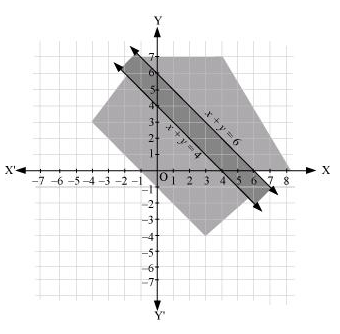# Solve the following system of inequalities graphically: x + y ≤ 6, x + y ≥ 4Question:

Solve the following system of inequalities graphically: x + y  6, x + y  4

Solution:

$x+y \leq 6$

$x+y \geq 4$

The graph of the lines, $x+y=6$ and $x+y=4$, are drawn in the figure below.

Inequality (1) represents the region below the line, $x+y=6$ (including the line $x+y=6$ ), and inequality (2) represents the region above the line, $x+y=4$ (including the line $x$ $+y=4$ ).

Hence, the solution of the given system of linear inequalities is represented by the common shaded region including the points on the respective lines as follows.